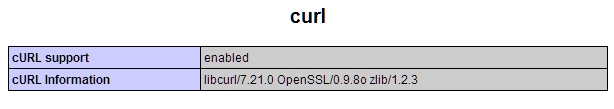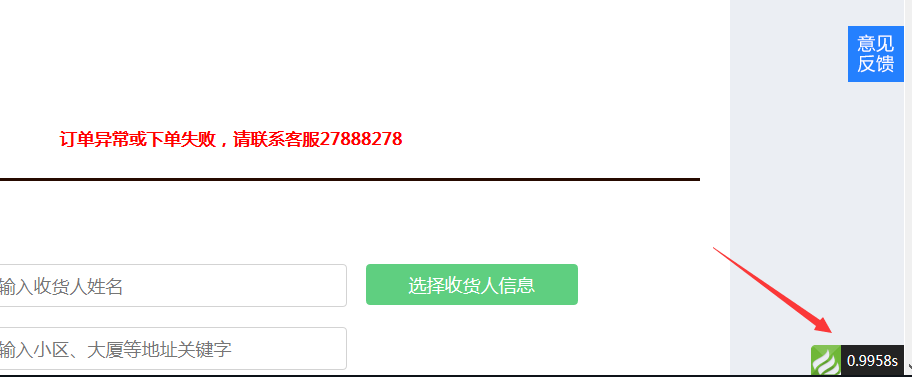# php的cURL库介绍

+关注继续查看

PHP cURL post get init exec cookie https

cURL 是一个利用URL语法规定来传输文件和数据的工具，支持很多协议，如HTTP、FTP、TELNET等。很多小偷程序都是使用这个函数。

cURL 是一种功能强大的库，支持很多不同的协议、选项，能提供 URL 请求相关的各种细节信息。

﹤?php
phpinfo();
?﹥//取消下面的注释
extension=php_curl.dll

$content = file_get_contents("http://www.doucube.com"); // or$lines = file("http://www.doucube.com");
// or
readfile(http://www.doucube.com);

// 1. 初始化
$ch = curl_init(); // 2. 设置选项，包括URL curl_setopt($ch, CURLOPT_URL, "http://www.doucube.com");
curl_setopt($ch, CURLOPT_RETURNTRANSFER, 1); curl_setopt($ch, CURLOPT_HEADER, 0);
// 3. 执行并获取HTML文档内容
$output = curl_exec($ch);
// 4. 释放curl句柄
curl_close($ch); 第二步（也就是 curl_setopt() ）最为重要，一切玄妙均在此。有一长串cURL参数可供设置，它们能指定URL请求的各个细节。要一次性全部看完并理解可能比较困难，所以今天我们只试一下那些更常用也更有用的选项。 检查错误 你可以加一段检查错误的语句（虽然这并不是必需的）： // ...$output = curl_exec($ch); if ($output === FALSE) {
echo "cURL Error: " . curl_error($ch); } // ... 请注意，比较的时候我们用的是“=== FALSE”，而非“== FALSE”。因为我们得区分 空输出 和 布尔值FALSE，后者才是真正的错误。 获取信息 这是另一个可选的设置项，能够在cURL执行后获取这一请求的有关信息： // ... curl_exec($ch);
$info = curl_getinfo($ch);
echo '获取'. $info['url'] . '耗时'.$info['total_time'] . '秒';
// ...

“url” //资源网络地址
“content_type” //内容编码
“http_code” //HTTP状态码
“request_size” //请求的大小
“filetime” //文件创建时间
“ssl_verify_result” //SSL验证结果
“redirect_count” //跳转技术
“total_time” //总耗时
“namelookup_time” //DNS查询耗时
“connect_time” //等待连接耗时
“pretransfer_time” //传输前准备耗时
“starttransfer_time” //开始传输的时间
“redirect_time”//重定向耗时 

// 测试用的URL
$urls = array( "http://www.doucube.com", "http://www.mozilla.com", "http://www.facebook.com" ); // 测试用的浏览器信息$browsers = array(
"standard" => array (
"user_agent" => "Mozilla/5.0 (Windows; U; Windows NT 6.1; en-US; rv:1.9.1.6) Gecko/20091201 Firefox/3.5.6 (.NET CLR 3.5.30729)",
"language" => "en-us,en;q=0.5"
),
"iphone" => array (
"user_agent" => "Mozilla/5.0 (iPhone; U; CPU like Mac OS X; en) AppleWebKit/420+ (KHTML, like Gecko) Version/3.0 Mobile/1A537a Safari/419.3",
"language" => "en"
),
"french" => array (
"user_agent" => "Mozilla/4.0 (compatible; MSIE 7.0; Windows NT 5.1; GTB6; .NET CLR 2.0.50727)",
"language" => "fr,fr-FR;q=0.5"
)
);
foreach ($urls as$url) {
echo "URL: $url\n"; foreach ($browsers as $test_name =>$browser) {
$ch = curl_init(); // 设置 url curl_setopt($ch, CURLOPT_URL, $url); // 设置浏览器的特定header curl_setopt($ch, CURLOPT_HTTPHEADER, array(
"User-Agent: {$browser['user_agent']}", "Accept-Language: {$browser['language']}"
));
// 页面内容我们并不需要
curl_setopt($ch, CURLOPT_NOBODY, 1); // 只需返回HTTP header curl_setopt($ch, CURLOPT_HEADER, 1);
// 返回结果，而不是输出它
curl_setopt($ch, CURLOPT_RETURNTRANSFER, 1);$output = curl_exec($ch); curl_close($ch);
// 有重定向的HTTP头信息吗?
if (preg_match("!Location: (.*)!", $output,$matches)) {
echo "$test_name: redirects to$matches\n";
} else {
echo "$test_name: no redirection\n"; } } echo "\n\n"; } 首先，我们建立一组需要测试的URL，接着指定一组需要测试的浏览器信息。最后通过循环测试各种URL和浏览器匹配可能产生的情况。 因为我们指定了cURL选项，所以返回的输出内容则只包括HTTP头信息（被存放于$output 中）。利用一个简单的正则，我们检查这个头信息中是否包含了“Location:”字样。

http://codeigniter.com/forums/do_search/

print_r($_POST); 接下来，写一段PHP脚本来执行cURL请求： $url = "http://localhost/post_output.php";
$post_data = array ( "foo" => "bar", "query" => "Nettuts", "action" => "Submit" );$ch = curl_init();
curl_setopt($ch, CURLOPT_URL,$url);
curl_setopt($ch, CURLOPT_RETURNTRANSFER, 1); // 我们在POST数据哦！ curl_setopt($ch, CURLOPT_POST, 1);
// 把post的变量加上
curl_setopt($ch, CURLOPT_POSTFIELDS,$post_data);
$output = curl_exec($ch);
curl_close($ch); echo$output;

$url = "http://localhost/upload_output.php";$post_data = array (
"foo" => "bar",
// 要上传的本地文件地址
);
$ch = curl_init(); curl_setopt($ch, CURLOPT_URL, $url); curl_setopt($ch, CURLOPT_RETURNTRANSFER, 1);
curl_setopt($ch, CURLOPT_POST, 1); curl_setopt($ch, CURLOPT_POSTFIELDS, $post_data);$output = curl_exec($ch); curl_close($ch);
echo $output; 如果你需要上传一个文件，只需要把文件路径像一个post变量一样传过去，不过记得在前面加上@符号。 cURL批处理（multi cURL） cURL还有一个高级特性——批处理句柄（handle）。这一特性允许你同时或异步地打开多个URL连接。 下面是来自来自php.net的示例代码： // 创建两个cURL资源$ch1 = curl_init();
$ch2 = curl_init(); // 指定URL和适当的参数 curl_setopt($ch1, CURLOPT_URL, "http://lxr.php.net/");
curl_setopt($ch1, CURLOPT_HEADER, 0); curl_setopt($ch2, CURLOPT_URL, "http://www.php.net/");
curl_setopt($ch2, CURLOPT_HEADER, 0); // 创建cURL批处理句柄$mh = curl_multi_init();
// 加上前面两个资源句柄
curl_multi_add_handle($mh,$ch1);
curl_multi_add_handle($mh,$ch2);
// 预定义一个状态变量
$active = null; // 执行批处理 do {$mrc = curl_multi_exec($mh,$active);
} while ($mrc == CURLM_CALL_MULTI_PERFORM); while ($active && $mrc == CURLM_OK) { if (curl_multi_select($mh) != -1) {
do {
$mrc = curl_multi_exec($mh, $active); } while ($mrc == CURLM_CALL_MULTI_PERFORM);
}
}
// 关闭各个句柄
curl_multi_remove_handle($mh,$ch1);
curl_multi_remove_handle($mh,$ch2);
curl_multi_close($mh); 这里要做的就是打开多个cURL句柄并指派给一个批处理句柄。然后你就只需在一个while循环里等它执行完毕。 这个示例中有两个主要循环。第一个 do-while 循环重复调用 curl_multi_exec() 。这个函数是无隔断（non-blocking）的，但会尽可能少地执行。它返回一个状态值，只要这个值等于常量 CURLM_CALL_MULTI_PERFORM ，就代表还有一些刻不容缓的工作要做（例如，把对应URL的http头信息发送出去）。也就是说，我们需要不断调用该函数，直到返回值发生改变。 而接下来的 while 循环，只在$active 变量为 true 时继续。这一变量之前作为第二个参数传给了 curl_multi_exec() ，代表只要批处理句柄中是否还有活动连接。接着，我们调用 curl_multi_select() ，在活动连接（例如接受服务器响应）出现之前，它都是被“屏蔽”的。这个函数成功执行后，我们又会进入另一个 do-while 循环，继续下一条URL。

WordPress 连接检查器

// CONFIG
$db_host = 'localhost';$db_user = 'root';
$db_pass = '';$db_name = 'wordpress';
$excluded_domains = array( 'localhost', 'www.mydomain.com');$max_connections = 10;
// 初始化一些变量
$url_list = array();$working_urls = array();
$dead_urls = array();$not_found_urls = array();
$active = null; // 连到 MySQL if (!mysql_connect($db_host, $db_user,$db_pass)) {
die('Could not connect: ' . mysql_error());
}
if (!mysql_select_db($db_name)) { die('Could not select db: ' . mysql_error()); } // 找出所有含有链接的文章$q = "SELECT post_content FROM wp_posts
WHERE post_content LIKE '%href=%'
AND post_status = 'publish'
AND post_type = 'post'";
$r = mysql_query($q) or die(mysql_error());
while ($d = mysql_fetch_assoc($r)) {
// 用正则匹配链接
if (preg_match_all("!href=\"(.*?)\"!", $d['post_content'],$matches)) {
foreach ($matches as$url) {
// exclude some domains
$tmp = parse_url($url);
if (in_array($tmp['host'],$excluded_domains)) {
continue;
}
// store the url
$url_list []=$url;
}
}
}
// 移除重复链接
$url_list = array_values(array_unique($url_list));
if (!$url_list) { die('No URL to check'); } 我们首先配置好数据库，一系列要排除的域名（$excluded_domains），以及最大并发连接数（$max_connections）。然后，连接数据库，获取文章和包含的链接，把它们收集到一个数组中（$url_list）。

// 1. 批处理器
$mh = curl_multi_init(); // 2. 加入需批量处理的URL for ($i = 0; $i <$max_connections; $i++) { add_url_to_multi_handle($mh, $url_list); } // 3. 初始处理 do {$mrc = curl_multi_exec($mh,$active);
} while ($mrc == CURLM_CALL_MULTI_PERFORM); // 4. 主循环 while ($active && $mrc == CURLM_OK) { // 5. 有活动连接 if (curl_multi_select($mh) != -1) {
// 6. 干活
do {
$mrc = curl_multi_exec($mh, $active); } while ($mrc == CURLM_CALL_MULTI_PERFORM);
// 7. 有信息否？
if ($mhinfo = curl_multi_info_read($mh)) {
// 意味着该连接正常结束
// 8. 从curl句柄获取信息
$chinfo = curl_getinfo($mhinfo['handle']);
// 9. 死链么？
if (!$chinfo['http_code']) {$dead_urls []= $chinfo['url']; // 10. 404了? } else if ($chinfo['http_code'] == 404) {
$not_found_urls []=$chinfo['url'];
// 11. 还能用
} else {
$working_urls []=$chinfo['url'];
}
// 12. 移除句柄
curl_multi_remove_handle($mh,$mhinfo['handle']);
curl_close($mhinfo['handle']); // 13. 加入新URL，干活 if (add_url_to_multi_handle($mh, $url_list)) { do {$mrc = curl_multi_exec($mh,$active);
} while ($mrc == CURLM_CALL_MULTI_PERFORM); } } } } // 14. 完了 curl_multi_close($mh);
echo implode("\n",$dead_urls) . "\n\n"; echo "==404 URLs==\n"; echo implode("\n",$not_found_urls) . "\n\n";
echo "==Working URLs==\n";
echo implode("\n",$working_urls); // 15. 向批处理器添加url function add_url_to_multi_handle($mh, $url_list) { static$index = 0;
// 如果还剩url没用
if ($url_list[$index]) {
// 新建curl句柄
$ch = curl_init(); // 配置url curl_setopt($ch, CURLOPT_URL, $url_list[$index]);
// 不想输出返回的内容
curl_setopt($ch, CURLOPT_RETURNTRANSFER, 1); // 重定向到哪儿我们就去哪儿 curl_setopt($ch, CURLOPT_FOLLOWLOCATION, 1);
// 不需要内容体，能够节约带宽和时间
curl_setopt($ch, CURLOPT_NOBODY, 1); // 加入到批处理器中 curl_multi_add_handle($mh, $ch); // 拨一下计数器，下次调用该函数就能添加下一个url了$index++;
return true;
} else {
// 没有新的URL需要处理了
return false;
}
}

HTTP 认证

$url = "http://www.somesite.com/members/";$ch = curl_init();
curl_setopt($ch, CURLOPT_URL,$url);
curl_setopt($ch, CURLOPT_RETURNTRANSFER, 1); // 发送用户名和密码 curl_setopt($ch, CURLOPT_USERPWD, "myusername:mypassword");
// 你可以允许其重定向
curl_setopt($ch, CURLOPT_FOLLOWLOCATION, 1); // 下面的选项让 cURL 在重定向后 // 也能发送用户名和密码 curl_setopt($ch, CURLOPT_UNRESTRICTED_AUTH, 1);
$output = curl_exec($ch);
curl_close($ch); FTP 上传 PHP 自带有 FTP 类库， 但你也能用 cURL： // 开一个文件指针$file = fopen("/path/to/file", "r");
// url里包含了大部分所需信息
$url = "ftp://username:password@mydomain.com:21/path/to/new/file";$ch = curl_init();
curl_setopt($ch, CURLOPT_URL,$url);
curl_setopt($ch, CURLOPT_RETURNTRANSFER, 1); // 上传相关的选项 curl_setopt($ch, CURLOPT_UPLOAD, 1);
curl_setopt($ch, CURLOPT_INFILE,$fp);
curl_setopt($ch, CURLOPT_INFILESIZE, filesize("/path/to/file")); // 是否开启ASCII模式 (上传文本文件时有用) curl_setopt($ch, CURLOPT_FTPASCII, 1);
$output = curl_exec($ch);
curl_close($ch); FQ术 你可以用代理发起cURL请求： $ch = curl_init();
curl_setopt($ch, CURLOPT_URL,'http://www.example.com'); curl_setopt($ch, CURLOPT_RETURNTRANSFER, 1);
// 指定代理地址
curl_setopt($ch, CURLOPT_PROXY, '11.11.11.11:8080'); // 如果需要的话，提供用户名和密码 curl_setopt($ch, CURLOPT_PROXYUSERPWD,'user:pass');
$output = curl_exec($ch);
curl_close ($ch); 回调函数 可以在一个URL请求过程中，让cURL调用某指定的回调函数。例如，在内容或者响应下载的过程中立刻开始利用数据，而不用等到完全下载完。 $ch = curl_init();
curl_setopt($ch, CURLOPT_URL,'http://api.doucube.com'); curl_setopt($ch, CURLOPT_WRITEFUNCTION,"progress_function");
curl_exec($ch); curl_close ($ch);
function progress_function($ch,$str) {
echo $str; return strlen($str);
}

POST数据

﹤?php
$phoneNumber = '13912345678';$message = 'This message was generated by curl and php';
$curlPost = 'pNUMBER=' . urlencode($phoneNumber) . '&MESSAGE=' . urlencode($message) . '&SUBMIT=Send';$ch = curl_init();
curl_setopt($ch, CURLOPT_URL, 'http://www.example.com/sendSMS.php'); curl_setopt($ch, CURLOPT_HEADER, 1);
curl_setopt($ch, CURLOPT_RETURNTRANSFER, 1); curl_setopt($ch, CURLOPT_POST, 1);
curl_setopt($ch, CURLOPT_POSTFIELDS,$curlPost);
$data = curl_exec(); curl_close($ch);
?﹥

﹤?php
$ch = curl_init(); curl_setopt($ch, CURLOPT_URL, 'http://www.example.com');
curl_setopt($ch, CURLOPT_HEADER, 1); curl_setopt($ch, CURLOPT_RETURNTRANSFER, 1);
curl_setopt($ch, CURLOPT_HTTPPROXYTUNNEL, 1); curl_setopt($ch, CURLOPT_PROXY, 'fakeproxy.com:1080');
curl_setopt($ch, CURLOPT_PROXYUSERPWD, 'user:password');$data = curl_exec();
curl_close($ch); ?﹥ SSL和Cookie 关于SSL也就是HTTPS协议，你只需要把CURLOPT_URL连接中的http://变成https://就可以了。当然，还有一个参数叫CURLOPT_SSL_VERIFYHOST可以设置为验证站点。 关于Cookie，你需要了解下面三个参数： CURLOPT_COOKIE，在当面的会话中设置一个cookie CURLOPT_COOKIEJAR，当会话结束的时候保存一个Cookie CURLOPT_COOKIEFILE，Cookie的文件。 HTTP服务器认证 最后，我们来看一看HTTP服务器认证的情况。 ﹤?php$ch = curl_init();
curl_setopt($ch, CURLOPT_URL, 'http://www.example.com'); curl_setopt($ch, CURLOPT_RETURNTRANSFER, 1);
curl_setopt($ch, CURLOPT_HTTPAUTH, CURLAUTH_BASIC); curl_setopt(CURLOPT_USERPWD, '[username]:[password]')$data = curl_exec();
curl_close(\$ch);
?﹥【解决方案】PHP使用CURL请求时，遇到name lookup timed out 如何解决
【解决方案】PHP使用CURL请求时，遇到name lookup timed out 如何解决
71 0php 使用 curl 库进行 ssl 双向认证
php 使用 curl 库进行 ssl 双向认证
132 033 0php使用CURL模拟POST请求函数
php使用CURL模拟POST请求函数
49 0php curl指定ip,php curl请求忽略本地host文件,php curl请求跳过本地host文件
php curl指定ip,php curl请求忽略本地host文件,php curl请求跳过本地host文件
82 0PHP：curl发送GET、POST请求
PHP：curl发送GET、POST请求
75 0【PHP】curl请求
【PHP】curl请求
132 0PHP curl报错"Problem (2) in the Chunked-Encoded data"解决方案
PHP curl报错"Problem (2) in the Chunked-Encoded data"解决方案
160 0php的file_get_contents和curl差距
php的file_get_contents和curl差距
89 0PHP curl 得到返回值且不显示在页面上
PHP curl 得到返回值且不显示在页面上
262 0

CSDN博客技术专家。已出版《微信公众平台开发最佳实践》第一版、第二版及第三版，《企业微信公众平台开发实战》等书籍。

PHP完全自学手册文档教程946088

PHP 2017.北京 全球开发者大会——高可用的PHP